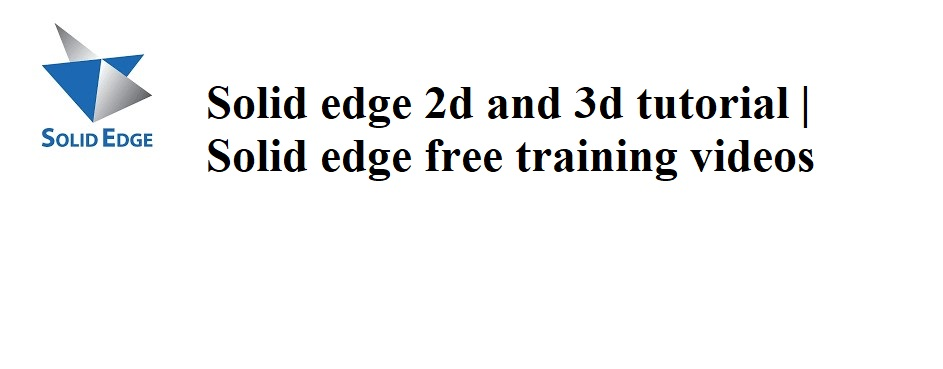October 25, 2021# Solid edge 2d and 3d tutorial | Solid edge free training videos

## Highlights

1.Solid edge 2d tutorial.

2.Solid edge 3d tutorial.

3.Solid edge 2d drafting tutorial.

1.Solid edge interview questions and answers.

2.Mechanical design engineer interview questions and answers for freshers.

3.Mechanical engineering interview questions and answers for freshers.

4.Top 30 hr interview questions and answers for freshers all jobs.

5.How to select right bearing for your application.

6.Design of mechanical shaft procedure.

## # Solid edge 2d tutorials

### Solid edge Part Modeling tutorial

##### 19.solid edge patterns tutorials.

20.solid edge sweep tutorials.

21. solid edge loft tutorials.

22.solid edge helix tutorials | Helix in solid edge.

23.solid edge sweep cut tutorials.

24.solid edge loft cut tutorials for beginners.

25.solid edge helix cut tutorials.

26. solid edge mounting boss tutorial.

27. solid edge lip tutorial 2021.

28.solid edge vent tutorials || HOW TO USE VENT IN SOLID EDGE || VENT IN SOLID EDGE.

29.solid edge web network |HOW TO USE WEB NETWORK IN SOLID EDGE.

30.solid edge rib tutorials #RIB.

31. solid edge thin wall tutorials #THIN WALL.

32.solid edge mirror part or feature | MIRROR PART OR FEATURE.

33. SOLID EDGE TUTORIALS | MOVE FACES | ROTATE FACES | OFFSET FACES.

34. SOLID EDGE TUTORIAL ADDITION OF PARALLEL PLANE, ANGLED PLANE, PERPENDICULAR AND COINCIDENT PLANE.

35.SOLID EDGE TUTORIAL SECTION IN PART OR ASSEMBLY.

36. solid edge apply material and find properties.

37. solid edge part modelling practice tutorials for beginners.

38.solid edge part modelling practice tutorials for beginners 2021.

39. solid edge part modelling practice tutorials for beginners | design of auxiliary fork.

40.solid edge part modelling practice tutorials for beginners 2021 | design of castle nut.

41.solid edge part modelling practice tutorials 2021 |Design of socket head Allen bolt.

42.solid edge part modelling practice tutorials for beginners.

43.solid edge part modelling tutorials for beginners 2021 | Design of twist drill bit.

44.solid edge apply color to part tutorial for beginners | How to apply color to part in solidedge.

45.solid edge assembly basics for beginners | How to get started in solid edge assembly 2021.

46. solid edge angle mate | how to use angle mate in solid edge assembly 2021.

47.solid edge path mate in assembly |How to use path mate in solid edge assembly.

48.solid edge part modelling practice tutorials for beginners 2021.

49.solid edge part modelling practice tutorials for beginners 2021.

50. PRESS TOOL ASSEMBLY PARTS MODELLING AND ASSEMBLY | SOLID EDGE ASSEMBLY TUTORIAL FOR BEGINNERS.

51.Universal joint assembly | Universal joint part modelling and assembly in solid edge.

52.solid edge text on surface tutorials | create text on surface in solidedge st10.

53.solid edge part modelling tutorials for beginners 2021| Circlip design in solid edge st10.

54.solid edge gears generation | how to create spur gear in solid edge st 10| Design of spur gear.

55.solid edge tutorial addition of logo | adding of photos or logo in solid edge st10.

56. Solid edge sheet metal contour flange.

57.solid edge tutorial sheet metal loft.

58.solid edge sheet metal hem | How to use solid edge sheet metal hem | Solid edge hem command.

59.Solid edge tutorial sheet metal Dimple | Dimple in solid edge.

60.Double bearing assembly parts design and assembly in solid edge | solid edge assembly tutorial.

61.Solid edge tutorial sheet metal louver | louver in solid edge.

62.how to flatten a sheet metal part in solid edge | solid edge sheet metal flatten.

63.solid edge tutorial drawn cutout | How to make drawn cut out in solid edge.

64.How to convert sheet metal to part in solid edge.

65.Helical gear generation in solid edge | How to create helical gear in solid edge.

66.Solid edge tutorial Knurling | Creating knurling in Solid edge st10.

67.How to replace part in solid edge assembly | Solid edge assembly tutorial.#### SKY HIGH eLearn

View all posts by SKY HIGH eLearn →

## 2 thoughts on “Solid edge 2d and 3d tutorial | Solid edge free training videos”

1.SANGEETHA R says: